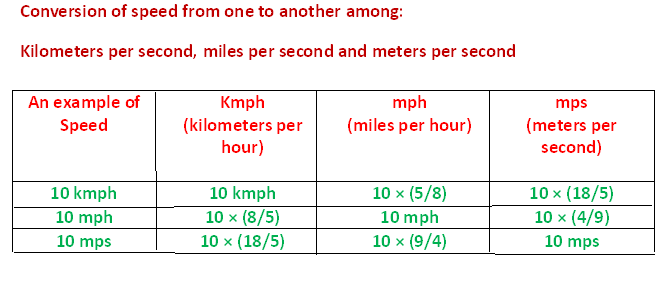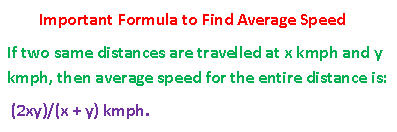## Time and Distance

Let d, s and t denote distance, speed and time respectively. Then,

Distance is product of speed and time, i.e.

1. d = s × t,

in which d is distance, s is speed and t is time.

Example:

The distance travelled by a bus running at an average speed 50 mph for 4 hours is

50 × 4 = 200 miles.

Similarly, speed is distance over time, i.e.:

2. s = d/t

The average speed of a car that has travelled:

100 miles in 2 hours is 50 mph (miles per hour)

Again, time is distance over speed, i.e.

3. t = d/s

Example:

The time a car will take to cover 100 miles at an average speed of 40 mph is

100/40 = 2.5 hours.

4. Conversion of Units:

1 kilometer = 1000 meters and 1 hour = 3600 seconds.

Therefore:

1km/hr = 1000m/3600s = 5/18 m/s or 5/18 ms-1

{Km/hr is read as kilometers per hour and m/s is read as meters per second)

Example:

The speed of a train is 190 km/hr. find the speed in m/s (i.e. meters per second)

Solution: 180 km/hr = 180 × (5/18) m/s = (180 × 5)/18 = 10 × 5 = 50 m/s

5. Similarly, as in point no. 4 above:

1 m/s = 18/5 km/hr

1 mph = 8/5 kmph

{mph is miles per hour and kmph is kilometers per hour and 1 mile is 8/5 kilometer}Example:

A city bus travels at an average speed of 20 m/s (20 meters per second). Express the speed in km/hr (kilometers per hour)

Solution:

20 m/s = 20 × 18/5 = (20 × 18)/5 = 4 × 18 = 72 km/hr.

Time and Speed are inversely proportional.

Consider two places separated by a distance of 100 miles.

a. a car will take 4 hours to cover 200 miles at an average speed 50 mph.

b. another car will take 5 hours to cover 200 miles at an average speed of 40 mph.

Ratio of speeds of the two cars = 50: 40 = 5: 4, and the

Ratio of times taken by the two cars = 4: 5, i.e. inverse of the ratio of speeds.

Two Very Important Rules on Time and Speed:

1. If speed increases K times, then new Time is T/K, where T is the original time taken to cover a same distance.

2. If the ratio of speeds of two cars is x: y, then the ratio of the times taken by them is y: x to cover a same distance

Example 1:

A car takes 9 hours to cover a distance of 500 miles. What time will it take to travel 500 miles if its speed grows 1.5 times the previous speed?

Solution: 1.5 = 3/2

If speed increases 1.5 times, i.e. 3/2 times, then time will decrease proportionately.

So, new time will be 2/3 times of previous time.

Therefore, new time taken is 9 × 2/3 = (9 × 2)/3 = 3 × 2 = 6 hours.

Example 2:

Driving at 5/6 th of his usual speed, John is late to his office by 15 mins. Find the usual time John takes to reach his office.

Solution:

Since, speed and time are inversely related, therefore

If new speed is 5/6 of original speed, then

New time is (6/5) normal time.

Since, John is late to the office by 15 minutes, therefore

(6/5)T ― T = 15, i.e. (1/5) T = 15 {T denotes normal time}

Therefore, T = 15 × 5 = 75 minutes.

So, john normally takes 75 minutes to reach his office.

Average Speed:

If two equal distances are travelled at speeds x mph and y mph, then the average speed over the entire distance isBut, average speed is not average of the two speeds, i.e average speed is NOT:

(x + y)/2

Example 1:

While going from A to B, the speed of a car is 40 mph and 60 mph while coming back from B to A, traveling the same distance. What is the average speed of the car over the entire distance?

Solution:

Since the distance traveled with different speeds is same, the average speed of the car over the entire distance is

(2 × 40 × 60)/ (40 + 60) = 4800/100 = 48

Therefore, average speed over the entire distance is 48 mph.

Note:  What Average Speed is NOT?

Average speed is not (40 + 60)/2 = 100/2 = 50 mph.

Average speed is not average of the two speeds, because time taken to travel each equal distance is different as speed is different.

Solved Examples on Time, Speed and Distance:

Example 1:

A car travels at a speed of 45 mph. What is the distance in meters the car will travel in 2 mins 30 seconds?

Solution:

45 mph = 45 × (8/5) kmph = (45 × 8)/5 = 72 kmph

72 kmph = 72 × 5/18 = (72 × 5)/18 = 20 mps {meters per second}

2 mins + 30 seconds is = 120 + 30 = 150 seconds.

Now, d = s × t,

Therefore, distance traveled in meters is:

20 × 150 = 3000 meters.

Example 2:

One car A completes one round of a circular path of radius 1.4 miles in 220 seconds, while another car B travels the diameter of the same path in 280 seconds. Find the ratio of the speeds of the two cars.

Solution:

Circumference of a circular path is 2∏r and since ∏ = 22/7

Speed of car A = d/t = (2 × ∏ × 1.4)/220 = (2 × (22/7) × 1.4)/220 = 0.04 miles per second.

Speed of car B = d/t = (2 × 1.4)/280 = 0.008

Therefore, the ratio of the speeds of the two cars is :

0.04: 0.008 = 5: 1